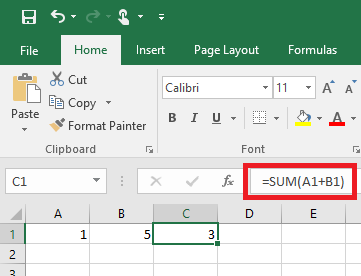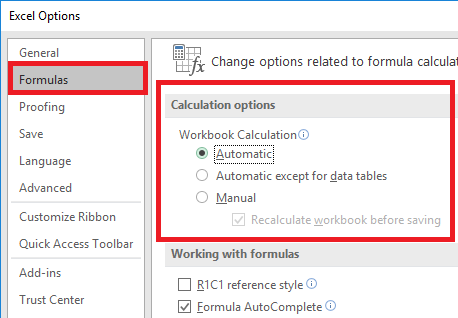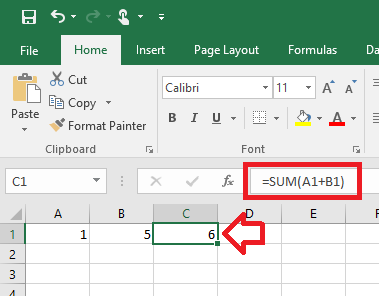# Excel 2016 – Auto-sum not working until edit formula or save spreadsheet

### Problem

When updating an Excel spreadsheet, formulas do not update automatically as values are entered or updates.

Instead – the formula only updates after the formula is edited or the spreadsheet is closed and opened again.### Solution

This happens when Excel has been configured to NOT update formulas automatically.

This setting applies to the program for the logged in user – so all spreadsheets will have the same issue.

The make Excel automatically calculate formulas again:

1. With Excel open, click on the ‘File’ menu
2. Then click on ‘Options’
3. In the left hand menu, select ‘Formulas’
4. Under ‘Calculation options’ select ‘Automatic’
5.6. Click ‘OK’ to save the changes.
7. All the formulas will now automatically update using the current values
8.## 8 comments on “Excel 2016 – Auto-sum not working until edit formula or save spreadsheet”

1. mehtab says:

thank you –

2. mithun says:

thanks man

3. Sayed Khan says:

thanks